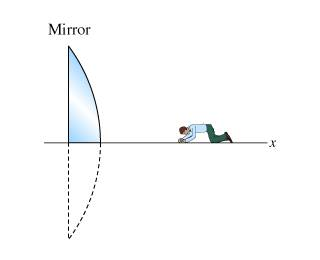# Image Size In A Mirror

## Homework Statement

A guy named Joe, who is 1.6 meters tall, enters a room in which someone has placed a large convex mirror with radius of curvature equal to 30 meters. The mirror has been cut in half, so that the axis of the mirror is at ground level. As Joe enters the room, he is 5 meters in front of the mirror, but he is looking the other way, so he fails to see it. When he turns around, he is startled by his own image in the mirror. ( i calculated 8.75m for this answer and its correct.)
NOW:
Joe is so startled by his image that he falls forward. (Assume that his feet stay at the same position.)

Now what is the length (i.e., the distance from head to toe) of Joe's image

1/f = 1/s' + 1/s

m = y'/y = s'/s

## The Attempt at a Solution

f = 15m

I calculated 1.2 and got this as a reply to my wrong answer:
This was Joe's height when standing. Since he has fallen, his length is now parallel to the axis of the mirror instead of perpendicular. Therefore, the normal magnification equation does not apply. See the hint for help

I now have two chances left for a correct answer. I do not know what I am doing wrong. I am sure I am just not thinking of something simple again.

here is picture:## The Attempt at a Solution

tiny-tim
Homework Helper
Hi jcvince17!
1/f = 1/s' + 1/s

Just use that equation twice: once for his feet, and once for his head.Hi jcvince17!

Just use that equation twice: once for his feet, and once for his head.doing so i get:

1/-15 = 1/s' + 1/3.4